## Maths icons

maths
maths
maths
maths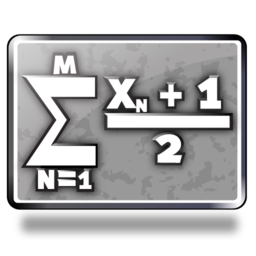maths
maths
maths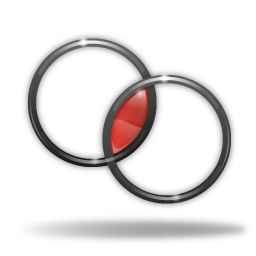maths
maths
maths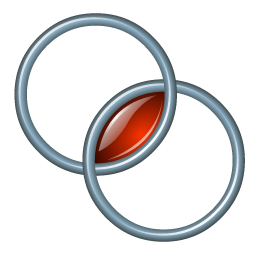maths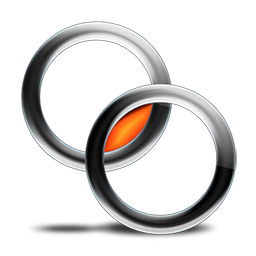mathsmathsmathsmaths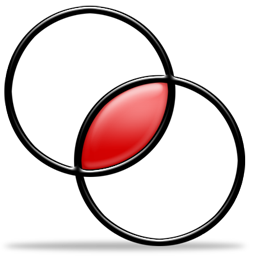maths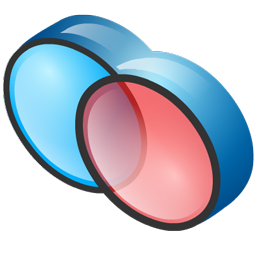mathsmaths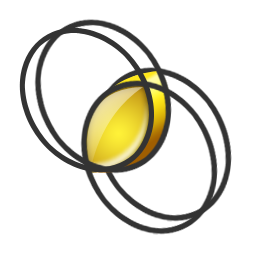maths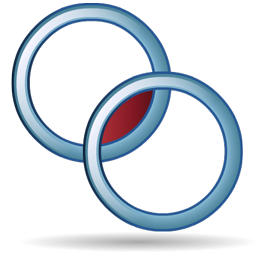maths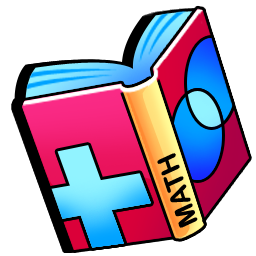maths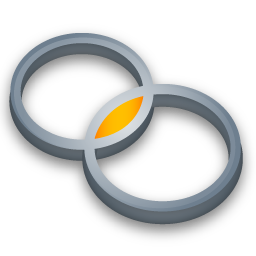maths

maths free icons and premium icon packs. Flat icons, Material icons, Glyph icons, iOS icons, Font icons, and more design styles. Vector files, including PNG and SVG icons. Ready for apps, web or social media projects.

maths
numbering
formula
equation
field numeric
field binary
computing
abacus
calculator

## Flat - Icons related to Maths

maths
numbering
formula
calculator buttons
field number
field binary
computing
abacus
calculator

## Material - Icons related to Maths

maths
numbering
formula
equation
field numeric
field binary
computing
abacusIcon name
Style name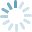### Color

This icon has not color editable properties, you can try with one of the following related:

### Related

Get our full library (2 million icons)

yearly subscription

Access to our full library of 2 million icons, 30 styles, 30 industries and growing

Download vector AI, SVG, PSD or Font source files of all our icons

Get all the files of the new icons we release: We add 100 new icons each week

Unlimited users

Great Value